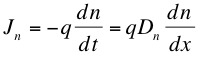# 5. Diffusion Current

We have modeled in the previous section a current based on the flow of charge carriers as the result of an electric field, or more adequately a potential difference. The potential gradient is what imparts a force on charged particles in the semiconductor. There is another current, however, which involves something called a concentration gradient, or differences in concentration from one place to another. This movement of carriers from one place to another according to random motion and statistical rules is called diffusion.Above: As with dye dropped into a liquid, electrons diffuse because of a concentration difference from place to place. In this example, the dye molecules spread throughout the water in a random fashion; statistically, they should! This is similar with electrons in a semiconductor, which should spread out over time until they reach an equilibrium (are uniformly spread).
Flowing in the direction of the negative gradient (derivative with respect to the x-direction, as that is sufficient for most semiconductor devices), we have that the diffusion current is proportional to the flow of carriers through a volume. Therefore, we can relate current density to the flow of charge concentration over time, as in the equation:where Jn is the current density, q is charge, and the change in n, the concentration of the charge carrier, over time is related to the change in n over distance by a diffusion constant Dn. In fact, this diffusion constant, like mobility from drift current, is derived statistically from the mean path length and lifetime of the carrier1, and can be obtained empirically. Furthermore, the same equation can be written for holes, but with opposite sign.
We can then sum up the components of current density in the following equations for both electrons and holes, including the drift current2:where μe and μh are the electron and hole mobilities respectively, E is the electric field strength, and n and p are the concentrations of electrons and holes respectively.
The diffusion and field currents are not independent of each other. We cannot see the connection between them in conductors, which have almost purely field currents because electrons freely move at all temperatures. This means that there is effectively no concentration gradient and therefore no diffusion current in conductors. However, in semiconductors the diffusion current and field current can actually cancel each other out, leading to effects we will study in P-N Junctions. Furthermore, the diffusion constant and mobility are connected by the Einstein relations, very useful parameters given below for both electrons and holes3.where k is Boltzmann’s constant, 1.38 x 10-23 Joules per Kelvin, and T is the temperature.

## References

1. Honsberg, Christiana, and Stuart Bowden. "Diffusion.” PVEducation.org. Web. 25 July 2012. <http://www.pveducation.org/pvcdrom/p...tion/diffusion>.
2. Goetzberger, Adolf et.al. Crystalline Silicon Solar Cells. Chichester: John Wiley & Sons Ltd., 1998.
3. Green, Martin A. Solar Cells: Operating Principles, Technology, and System Applications. Englewood Cliffs: Prentice-Hall, Inc., 1982. Full book ordering information at www.pv.unsw.edu.au.